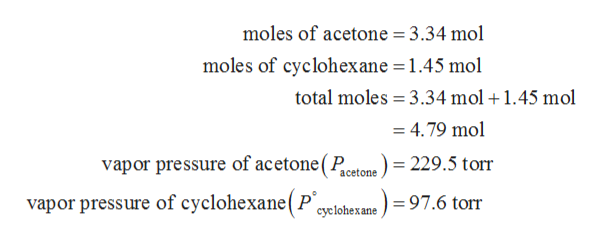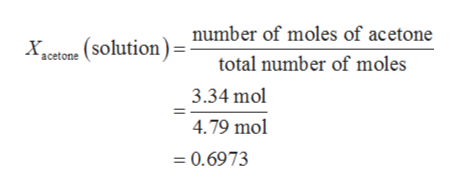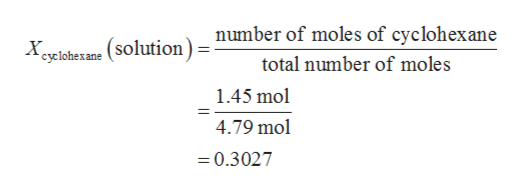# A solution is prepared by mixing 3.34 mol of acetone with 1.45 mol of cyclohexane at 30°C. Calculate the Xacetone and Xcyclohexane in the vapor above the solution.  P°acetone = 229.5 torr and P°cyclohexane = 97.6 torr.

Question
37 views

A solution is prepared by mixing 3.34 mol of acetone with 1.45 mol of cyclohexane at 30°C.

Calculate the Xacetone and Xcyclohexane in the vapor above the solution.
P°acetone = 229.5 torr and P°cyclohexane = 97.6 torr.

check_circle

Step 1

The values are given as follows:help_outlineImage Transcriptionclosemoles of acetone = 3.34 mol moles of cyclohexane = 1.45 mol - total moles 3.34 mol + 1.45 mol =4.79 mol vapor pressure of acetone(Ptone ) = 229.5 torr vapor pressure of cyclohexane(P cvelohexane ) =97.6 torr fullscreen
Step 2

The mole fraction of acetone in the solution can be calculated as follows:help_outlineImage Transcriptionclose(solution)= number of moles of acetone total number of moles х, aceton 3.34 mol 4.79 mol = 0.6973 fullscreen
Step 3

The mole fraction of cyclohexane in the sol...help_outlineImage Transcriptionclosenumber of moles of cyclohexane Xeyclohexane (Solution) total number of moles 1.45 mol 4.79 mol =0.3027 fullscreen

### Want to see the full answer?

See Solution

#### Want to see this answer and more?

Solutions are written by subject experts who are available 24/7. Questions are typically answered within 1 hour.*

See Solution
*Response times may vary by subject and question.
Tagged in

### Physical Chemistry